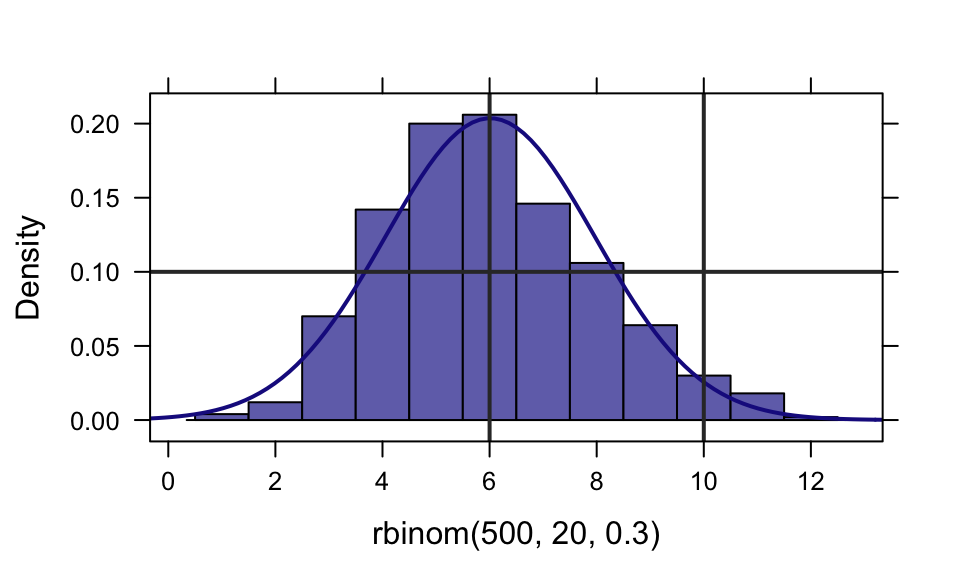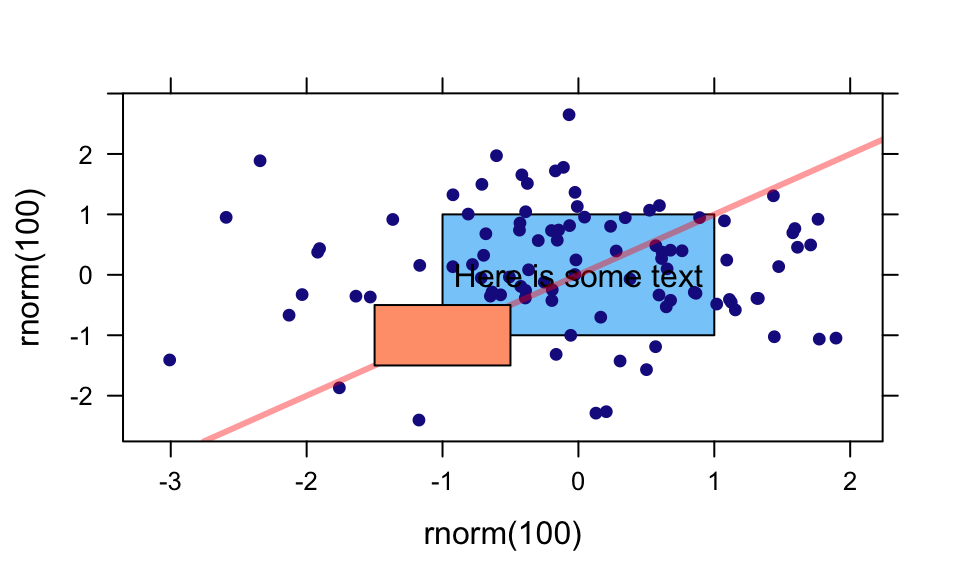# Graphics with the mosaic and lattice packages

### Randall Pruim

#### August, 2014

This vignette is simply a suite of plots that exist primarily as part of our quality control for the package. But since the examples might be useful to others as well, we’ve added this as a vignette in the package.

This way of doing this is largely superceded by our `ggformula` package which provides a formula interface to `ggplot2`. You might also like to see the vignette that compares using `lattice` to using `ggformula`.

### lattice extras

The `mosaic` package resets the default panel function for histograms. This changes the default for bin selection and provides some additional arguments to histogram.

``histogram(~ rbinom( 500, 20, .3), width=1, fit="normal", v=c(6,10), h=0.1 )```ladd()` provides a relatively easy way to add additional things to a lattice graphic.

``````xyplot( rnorm(100) ~ rnorm(100) )
ladd( grid.text("Here is some text", x=0, y=0, default.units="native") )
ladd( panel.abline( a=0, b=1, col="red", lwd=3, alpha=.4 ) )
ladd( panel.rect(x=-1, y=-1, width=1, height=1, col="gray80", fill="lightsalmon"))
ladd( panel.rect(x=0, y=0, width=2, height=2, col="gray80", fill="lightskyblue"),
under=TRUE)``````### mplot()

In addition to the interactive uses of `mplot()`, it can be used in place of `plot()` in several settings.

``````require(gridExtra)
mod <- lm(width ~ length * sex, data = KidsFeet)
mplot(mod, which = 1:7, multiplot = TRUE, ncol = 2)``````
``````## `geom_smooth()` using formula 'y ~ x'
## `geom_smooth()` using formula 'y ~ x'
## `geom_smooth()` using formula 'y ~ x'
## `geom_smooth()` using formula 'y ~ x'``````